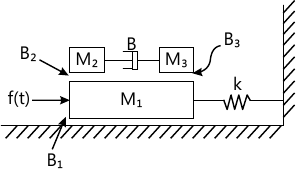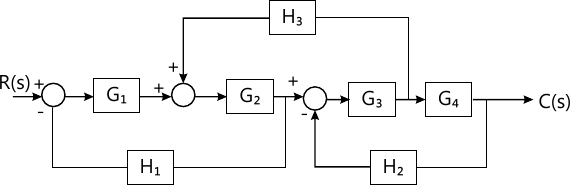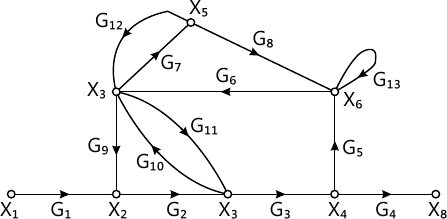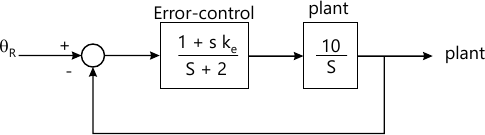Total marks: --
Total time: --
INSTRUCTIONS
(1) Assume appropriate data and state your reasons
(2) Marks are given to the right of every question
(3) Draw neat diagrams wherever necessary

1 (a) Compare the linear and non-linear control system.
4 M
1 (b) For the two port network shown below, obtain the transfer functions, $i) \ \dfrac {V_2(s)}{V_1(s)} \ and \ ii) \ \dfrac {V_1 (s)}{1_1(s)}$8 M
1 (c) For the rotational system shown in below, i) Draw the mechanical network ii) Write the differential equations iii) Obtain torque to voltage analogy.8 M

2 (a) Illustrate how to perform the following, in connection with block diagram reduction reules:
i) Shifting a take-off after a summing point.
Shifting a take-off point before a summing point.
4 M
2 (b) The performance equations of a controlled system are given by the following set of linear algebraic equations:
i) Draw the block diagram
ii) Find the overall transfer function C(s)/R(s) using block diagram reduction technique. $E_1(s)=R(s)-H_2(s)C(s); \ E_2(s)=E_1(s)-H_1(s)E_4(s); \ E_2(s)=G_1(8)E_2(8)-H_2(s)C(s) E_4(s)=G_2(s)E_2(s); \ C(s)=G_2(s)E_4(s)$
8 M
2 (c) Draw the corresponding signal flow graph for the given block diagram is shown in below, and obtain the overall transfer function by Mason's gain formula.8 M

3 (a) Derive the expression for peak time.
4 M
3 (b) The loop transfer function of a feed back control system is given by, $G(s)H(s)= \dfrac {100}{s^2(s+4)(s+12)}$ i) Determine the static error co-efficients
ii) Determine the steady state error for the input r(t)=2t2+5t+10
8 M
3 (c) A system is given by differential equation, $\dfrac {d^2 y(t)} {dt^2}+4\dfrac {dy(1)}{dt}+ 8y(t)=8x(t)$ Where y(t)=output and x(t)=input
Determine
i) Peak time
ii) Peak over shoot
iii) Settling time
iv) expression of the output response.
8 M

4 (a) Define the term stability applied to control system and what is the difference between absolute stability and relative stability.
4 M
4 (b) Using Routh's criterion determine the stability of following systems:
i) Its open loop transfer function has poles at s=0, s=-1, s=-3 and zero at s=-5. Gain K=10.
ii) It is a type one system with an error constant of 10 sec-1 and poles at s-3 and s=-6
8 M
4 (c) Using RH criterion determine the stability of the system having the characteristics equation, s4+10s2+36s2+70s+75=0 has roots more negative than s=-2.
8 M

5 (a) The open-loop transfer function of a feed back control system in $G(s)H(s)= \dfrac {K}{(s+1)(s+2)(s+3)}$ check whether the following points are on the root locus. IT so, find the value of K at these points, i) s=-1.5 ii) s=0.5+j2
6 M
5 (b) Sketch the root locus plot for a negative feed back control system characterized by an open loop transfer function, $G(s)H(s)= \dfrac {K}{s(s+3)(s^2+3s+11.2s)}$ Comment on stability.
14 M

6 (a) State the advantages and limitations of frequency domain approach.
6 M
6 (b) Determine the transfer function of a system whose asymptotic gain plot is shown in below.10 M
6 (c) List the effect of lead compensation.
4 M

7 (a) Draw polar plot of $G(s)H(s)= \dfrac {100}{(s+2)(s+4)(s+8)}$
4 M
7 (b) Explain Nyquist stability criterion.
6 M
7 (c) Sketch the Nyquist plot for, $GH(s)= \dfrac {k}{s(s+1)(s+2)}$ Then find the range of K for closed loop stability.
10 M

8 (a) Define the following terms: i) state ii) state variables iii) state space.
6 M
8 (b) List the advantages of state variable analysis.
4 M
8 (c) Obtain the state transition matrix for, $A=\begin{bmatrix} 0 &-1 \\2 &-3 \end{bmatrix}$
10 M

More question papers from Control Systems